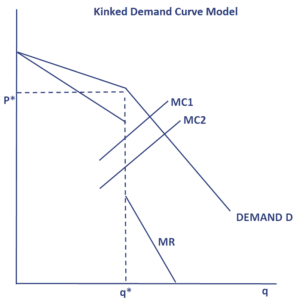# Kinked Demand Curve Model

The kinked demand curve model is a traditional oligopoly model. The model tries to explain how companies in an oligopoly market behave and react to each other’s behavior. On this page, we explain the kinked demand curve model, discuss the kinked demand curve graph, and discuss an example.

## Kinked demand curve explained

What is the kinked demand curve model? The kinked demand curve model for oligopoly markets is based on the assumption that companies within the market are interdependent. This means that the behavior of one company is expected to impact the behavior of the other companies in the market. In the case of the kinked demand curve model this interdepence works as follows.

The model assumes that an increase in a firm’s price will not be followed by a price increase by the other firms within the market. Conversely, a price decrease will lead to a reaction by the other companies. The consequence is that the firms have little to gain from a price increase as they will lose market share to the other companies that do not change their price. At the same time, decreasing the price will cause will cause revenues for all companies to go down simultaneously.

The following figure plots the kinked demand curve diagram.  Because of this kinked shape, the profit maximizing level of output is exactly at the kink. The most important consequence of this kinked shape is a “gap” in the marginal revenue curve, which is also shown in the plot.## Kinked demand curve limitations

In the above kinked demand curve example we put the kink at a certain price and quantity. In fact, this price and quantity are not a result of the model, but have been chosen arbitrarily. This illustrates the main shortcoming of the kinked demand curve model. The model is incomplete since it is not tell us what explains where the kink will be. This important element is outside the scope of the model.

## Summary

We explained the kinked demand curve model of oligopoly. While this is one the main oligopoly models used by economists to explain the behavior of firms within the monopoly, it has a serious flaw. In particular, it does not explain how the price is set. This is why other models have been proposed to explain how oligopolies might work (for example, the Stackelberg model).